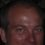# Integer Partitioning May Be Useful for Developing Statistical Models

I never studied number theory in school, so my approach to the problem may be a bit different.
I approached the problem by tabulating results and then searching for patterns. I never discovered an algebraic answer, but was able to develop a fractal approach to solving partition problems. That means that my answers are in terms of table coordinates. The partition table is easy to generate due to the fractal nature of the pattern. I've also learned how to answer the question of how many partitions may contain a subgroup of values or how many partitions that contain a given number of parts also contain certain values. I learned that I could partition non-integers, because if P(K) u=1 means the partition of K with one being the smallest value for any part, then P(K) u = P(cK) cu. This means that if u=0.1, I can find the answer by assigning c=10. In the practical world, all results are reported to a number of significant figures and data usually contains only positive values, so the number of ways to obtain a value in the practical world is actually finite, not infinite. If K is a mean value, all possible ways to obtain that mean can be found if we define the number of parts permitted and the number of significant figures. By looking at the frequency of parts with a given value, I can also graph the distribution of values without any restriction on standard deviation.Note by John Leach
3 years, 6 months ago

This discussion board is a place to discuss our Daily Challenges and the math and science related to those challenges. Explanations are more than just a solution — they should explain the steps and thinking strategies that you used to obtain the solution. Comments should further the discussion of math and science.

When posting on Brilliant:

• Use the emojis to react to an explanation, whether you're congratulating a job well done , or just really confused .
• Ask specific questions about the challenge or the steps in somebody's explanation. Well-posed questions can add a lot to the discussion, but posting "I don't understand!" doesn't help anyone.
• Try to contribute something new to the discussion, whether it is an extension, generalization or other idea related to the challenge.

MarkdownAppears as
*italics* or _italics_ italics
**bold** or __bold__ bold
- bulleted- list
• bulleted
• list
1. numbered2. list
1. numbered
2. list
Note: you must add a full line of space before and after lists for them to show up correctly
paragraph 1paragraph 2

paragraph 1

paragraph 2

[example link](https://brilliant.org)example link
> This is a quote
This is a quote
    # I indented these lines
# 4 spaces, and now they show
# up as a code block.

print "hello world"
# I indented these lines
# 4 spaces, and now they show
# up as a code block.

print "hello world"
MathAppears as
Remember to wrap math in $$ ... $$ or $ ... $ to ensure proper formatting.
2 \times 3 $2 \times 3$
2^{34} $2^{34}$
a_{i-1} $a_{i-1}$
\frac{2}{3} $\frac{2}{3}$
\sqrt{2} $\sqrt{2}$
\sum_{i=1}^3 $\sum_{i=1}^3$
\sin \theta $\sin \theta$
\boxed{123} $\boxed{123}$